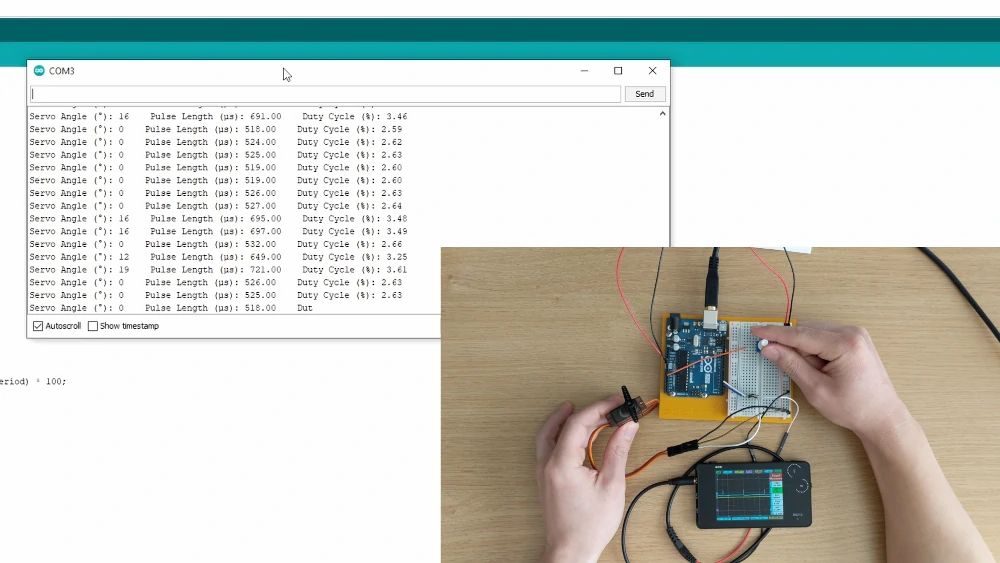Search
•Brandon

# How to Control a Servo with Arduino

In this post, I'm going to demonstrate how to control a servo with a potentiometer and an Arduino. I'm also going to discuss the type of signal that comes out of the Arduino to control the servo.

Parts Needed:

For this demonstration, you'll need:

All of which can be found in the Arduino starter kit. I also use a mini oscilloscope to view the signal coming out of the Arduino.

What is a Servo?

A servo is simply a brushed motor with built in gearbox, potentiometer, and motor controller. The motor controller controls the direction of the motor, and when it turns on an off, based on the signal it receives from the potentiometer and from its signal input cable.There are three leads to each servo. They are color coded where red is the input voltage, black or brown is ground, and white or yellow is the input signal.Pulse Width Modulation (PWM)

The servo is controlled by pulse width modulation, or PWM. This type of signal can be created using the digital pins on the Arduino. A PWM signal can be created with the analogWrite() command, just as I demonstrated in a previous entry, or the Servo.write() command. However, unlike the analogWrite() command that outputs a PWM signal at 490Hz, the servo expects a frequency of 50Hz. This is achieved with Arduino's servo library.At 50Hz, the time between pulses is 20ms, which is significantly greater than the time between pulses from a 490Hz PWM signal.

For a servo, it's generally assumed that the servo is expecting a pulse of about 1 to 2 milliseconds. This implies that at 1ms, the servo will be at 0 degrees, and at 2ms the servo will be at 180 degrees. However, in this demonstration, we'll take a look at the actual pulse lengths.Building the Circuit

The goal of this circuit is to read analog values coming from a potentiometer and using those values to directly control the angle of a servo. I'm also going to explore the PWM signal that the Arduino is creating for the servo.

• Start by wiring 5V and Ground to the potentiometer.

• Connect the potentiometer output to A0.

• Wire the servo to 5V and Ground, and the signal wire to pin 3.

• Then connect a jumper in pin 4 to read the signal coming out of pin 3.Arduino Code

In this example, I want to simply control the angle of the servo with the potentiometer. The code for this example can be found here.

• First include the servo library and define the pins for the potentiometer output, servo input, and signal being read from the servo output.

```#include <Servo.h>
#define SensorPin A0
#define ServoPin 3
#define PulsePin 4```
• I also define a servo object and integers for the servo angle and potentiometer value.

```Servo Servo1;
int pos = 0;
int SensorVal = 0;```
• Initialize a floating point variable for the pulse length, a constant float for the period of the PWM signal, and the duty cycle.

• The period is set to 0.02, because the period of a 50Hz PWM signal is 0.02 seconds.

```float PulseLength = 0.0;
const float Period = 0.02;
float DutyCycle = 0.0;```
• In the setup, attach the servo object to the servo pin.

• Set the pin reading the pulse from the servo pin to INPUT.

• Set the baud rate to 9600.

```  Servo1.attach(ServoPin);
pinMode(PulsePin, INPUT);
Serial.begin(9600);```
• In the loop, read the value of the potentiometer with the analogRead() command.

• Map this value from 0-1023 to 0-180.

• Then send the mapped value to the servo pin, using the Servo.write() command.

```  SensorVal = analogRead(SensorPin);
pos = map(SensorVal, 0, 1023, 0, 180);
Servo1.write(pos);```
• Measure the time that the PWM signal is in the HIGH state, by using the pulseIn() command. This outputs a number in microseconds.

`  PulseLength = pulseIn(PulsePin, HIGH);`
• Next to calculate the duty cycle by first converting the pulse length from microseconds to seconds.

• Then divide this value by the period and multiply it by 100.

`DutyCycle = ((PulseLength / pow(10,6)) / Period) * 100;`
• Printing the servo angle, pulse length and duty cycle.

```  Serial.print("Servo Angle (°): ");
Serial.print(pos);
Serial.print("    ");
Serial.print("Pulse Length (µs): ");
Serial.print(PulseLength);
Serial.print("    ");
Serial.print("Duty Cycle (%): ");
Serial.println(DutyCycle);```

After the upload, you should see the servo move between 0 and 180 degrees by moving the potentiometer to its end points.When the serial monitor is opened, the pulse lengths go beyond the expected 1-2ms when the servo is being moved to 0 and 180 degrees. At 0 degrees, the pulse length is actually about 0.5ms, and at 180 degrees, the pulse length is actually about 2.3ms.A 1ms pulse length is actually about 47 degrees and a 2ms pulse length is about 145 degrees. Try this out with your own servo and Arduino to see what you get.Powering the Servo

Sometimes, the servo can a bit jittery when it's being powered by the Arduino regulated output voltage. I've found that adding capacitors to the circuit can help with the problem, but I've had the best results by powering servo with a separate power source or voltage regulator. Just keep in mind the maximum voltage of your servo. Also, make sure to ground the power source to the Arduino.Thanks for following along. Check out early entries from this Introduction to Arduino Series. Subscribe below to stay up to date with future releases.

Also, if you're interested in the engineering design process and how to create a robotics prototype at home, check out my course.

The Bored Robot LLC is a participant in the Amazon Services LLC Associates Program, an affiliate advertising program designed to provide a means for sites to earn advertising fees by advertising and linking to Amazon.com.